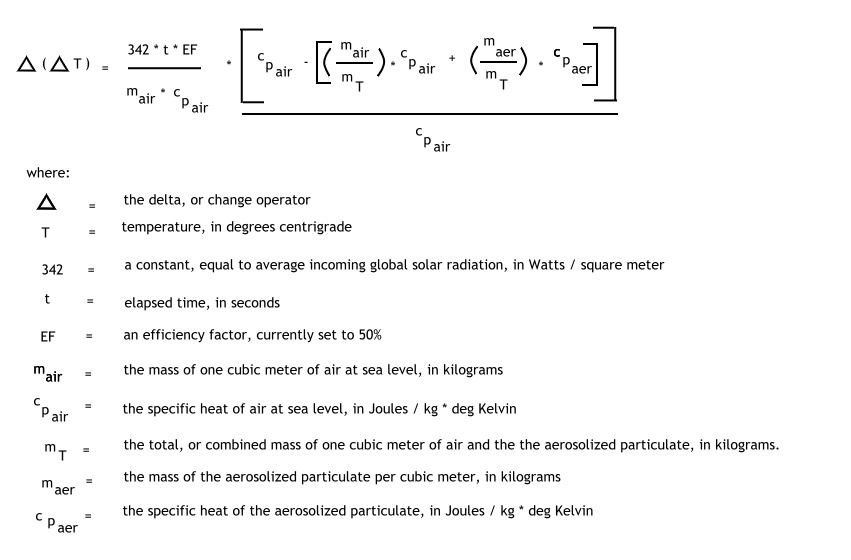A Global Warming Model
Part II
Clifford E Carnicom
Santa Fe, NM
Apr 10 2007

The details of the Global Warming Model  are presented on this page.

The model has the following final form:The model is developed in the following manner: (text form)

The definition of heat capacity is given as1

C = dQ/dT

which states that the heat capacity of a substance is defined as the instantaneous change in the quantity of heat (joules) with respect to an instantaneous change in temperature (degrees Kelvin or centigrade).  The units of C are J / K, or joules per degree Kelvin.

The specific heat capacity is furthermore defined as:2

c = del Q / (m * del T)

where Q is in joules, m is the mass in kilograms (kg) and T is in degrees Kelvin or centigrade, and del is the change operator.

Specific heats are measured values that are commonly available, and they indicate how much energy is required to raise a unit volume of material a unit rise in temperature (centrigrade or Kelvin).

Specific heats can be measured at constant pressure (cp) or constant volume (cv). Specific heats for gases do not vary significantly over large temperature variations3, and they may therefore usually be treated as constants.  A suitable value of cp for air is 1.003 kJ/ kg K4. For solids and liquids, the difference between cp and cv is usually quite small5 and can usually be ignored; values for cp are readily available.

As the definition of specific heat results from a differential form, this paper will focus on the change in a small volume of air, namely 1 cubic meter of air under ideal gas conditions.

The specific heat can be rearranged to:

del T = del Q /  ( m * cp )

this is equivalent to:

del T = ( Watts / m * cp ) * t

where t is time in seconds, and Watts is the incoming energy in joules /second.

The model under consideration examines the above change from a differential standpoint, i.e., what is the effect upon temperature change with respect to an incremental change in input energy for a unit mass of air?  The incremental change in input energy will result from the change in specific heat of a mixture, i.e, air vs. air with aerosolized particulates.  Developing further, our model now has the form:

del (del T) = ( t / m * cp) * del (Watts)

The model will also be permitted to include an efficiency factor (EF), as not all of the energy coming into the system (i.e., solar energy) will be absorbed.  A current estimate for this efficiency factor is set at 50 percent.6

or

del (del T) = ( EF  * t ) / ( m * cp ) * del ( Watts )

The next problem is to determine a value of cp for the modified atmosphere, i.,e. air with aerosolized particulates added to the cubic meter of air under examination.  The specific heat capacity of a mixture is given7 as:

cp(air+aerosol) = sum ( mfi * cpi)

where mfi is the mass fraction of the ith component of mixture, and cpi is the specific heat capacity of the ith component of the mixture.

mfi is defined as mi / m

whre mi is the mass of the ith component and m is the total mass of the mixture.

Let us now refer to:

mair = mass of 1 cubic meter of air in kg

maer = mass of aerosols added to 1 cubic meter of air in kg

cpair = specific heat of air in J /kg  K

cpaer = specific heat of aerosol in J /kg K

cp(air+aerosol) = [mair / (mair + maer) ] * cpair + [maer / (mair + maer)] * cpaer

It can be proposed that del (Watts) can be aequately represented by:

del (Watts ) = [ del (cp) / cpair ] * Average Solar Radiation

and that

del (cp) = cpair – cp(air+aerosol)

or that

del (del T) = [( EF  * t ) / ( mair * cpair )] *  [( cpair – cp(aer+aerosol) ) / cpair] * Average Solar Radiation

or that

del (del T) = [( EF  * t ) / ( mair * cpair )] *  [ cpair –   ( [mair / (mair + maer) ] * cpair + [maer / (mair + maer)] * cpaer  ) / cpair ] * Average Solar Radiation

which is equivalent to the model presented above.

The average incoming solar radiation (insolation) to the earth will be taken as 342 W / m2.8

The mass of air will be taken as 1.2 kg / m3.

The specific heat capacity of barium, cpaer, is .19 J / kg  K.9,10

The specific heat capacity of air, cpair,  is 1.003 J /kg K.

The efficiency factor is selected as .50.

In the model proposed, the mass of the aerosol varies from 0 to 50 ugms (micrograms) per cubic meter, or from 0 to 50E-9kg/ m3.

Time is measured in seconds, and varies from 0 to 50 years (one year = 31536000 seconds).

The model evaluated with respect to variations in time and mass concentration of the aerosol will produce the graphic result of this report.  The final units of the model are in degrees centigrade per m2, which corresponds to the differential element of air chosen as 1 cubic meter.  A more complete partial differential model of change with respect to both del (Watts) and del (cp)  may be pursued in the future if warranted. The model is not intended by any respects to be all inclusive of the global warming issue; it is intended to introduce, in a quantitative sense, the consideration of heating of the lower atmosphere from the artificial introduction of particulates.

References:

1. Walter Benenson, Handbook of Physics, (Springer-Verlag, 2002), 684.
2. Benenson, 687.
3. Merle C. Potter, Thermodynamics for Engineers, (McGraw-Hill, 1983), 55.
4. Potter, 289.
5. Potter, 56.
6. National Snow and Ice Data Center,  University of Colorado, Boulder,  Arctic Climate and Meteorology, http://nsidc.org/arcticmet/factors/radiation.html
7. Potter, 251.